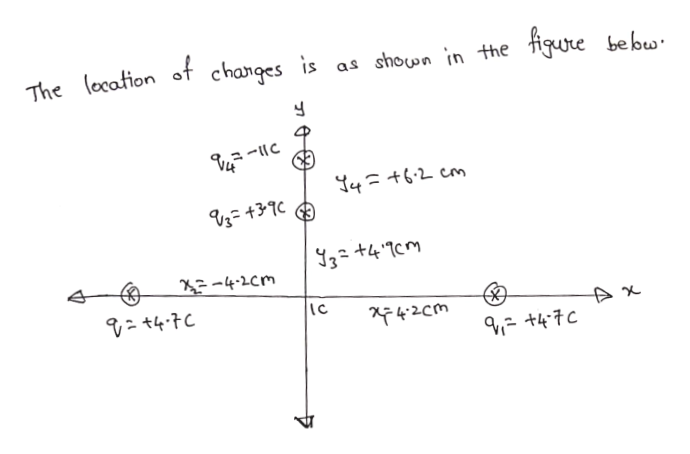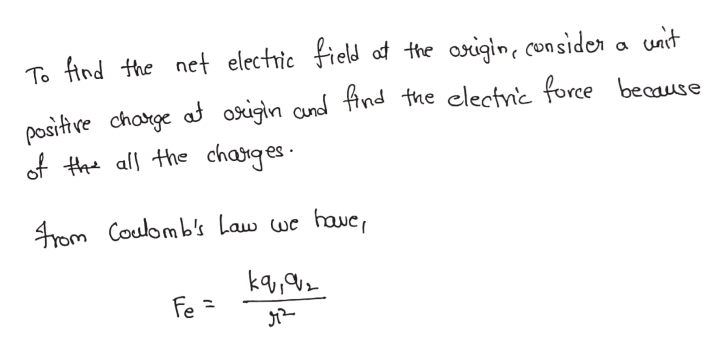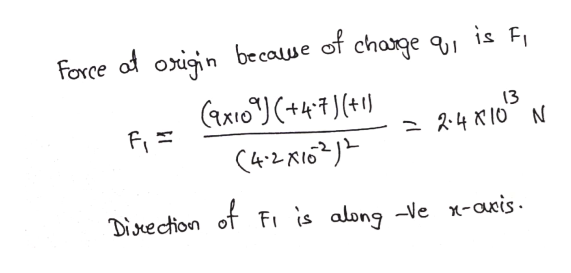Two charges are located on the x axis: q1 = +4.7C at x1 = +4.2 cm, and q2 = +4.7C at x2 = -4.2 cm. Two other charges are located on the y axis: q3 = +3.9C at y3 = +4.9 cm, and q4 = -11C at y4 = +6.2 cm. Find (a) the magnitude and (b) the direction of the net electric field at the origin.

Question

Two charges are located on the x axis: q1 = +4.7C at x1 = +4.2 cm, and q2 = +4.7C at x2 = -4.2 cm. Two other charges are located on the y axis: q3 = +3.9C at y3 = +4.9 cm, and q4 = -11C at y4 = +6.2 cm. Find (a) the magnitude and (b) the direction of the net electric field at the origin.

Step 1help_outlineImage TranscriptioncloseThe (ocation of changes is as shown 'n the figure bebw Y4ニ+62 cm 3-4CM Wつ7-カー そ42cm +47C fullscreen
Step 2help_outlineImage Transcriptioncloseunit To find the net electric field af the aigin, con sider positve chotge af osigln and find the clectnic force beaause of tall the charges 4rom Coulomb's Law wc havc, ka Fe = fullscreen
Step 3help_outlineImage TranscriptioncloseForce of oign becaue of chage 9, is F 13 2.4 K10 N Disuection of Fi is along -Ve x-axis. fullscreen

Want to see the full answer?

See Solution

Want to see this answer and more?

Our solutions are written by experts, many with advanced degrees, and available 24/7

See Solution
Tagged in

Physics